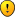## Mystery Numbers Killer

For fans of Killer Sudoku, Samurai Sudoku and other variants

### Mystery Numbers Killer

Rule : fill in the grid with all different positive integersEnjoyLast edited by Jean-Christophe on Thu Oct 05, 2006 3:02 am, edited 2 times in total.
Jean-Christophe

Posts: 149
Joined: 22 January 2006

Sorry, but I don't understand the rules.

Pyrrhon
Pyrrhon

Posts: 240
Joined: 26 April 2006

Rule:
Each row/column/box contains all elements from {A,B,C,D,E,F,G,H,J}, a set of 9 distinctive positive integers.
Your job is to determine the values of these 9 numbers and solve the grid.
udosuk

Posts: 2698
Joined: 17 July 2005

I though it was clear enough.
Anyhow, it's nine numbers out of the "natural numbers" : 1,2,3,4.... infinity
No zero, fractions, decimals..., just plain positive integers
And as you can see, these are not the traditional 1..9
"All different" means : numbers cannot be repeated, must be distinct.
It's a killer, so the cells in the cage must add up to the sum displayed. And no number may be repeated within a cage, even if it would be otherwise permitted by rules for regular sudoku.
It can be solved using logic. No need of guess or T&E.
BTW notice the void/empty cell in R5C5
Hint : Think in terms of "45". How many "45" make the whole puzzle ?
Jean-Christophe

Posts: 149
Joined: 22 January 2006

Thanks.

after udosuks explanation I was able to solve it.

My key steps were:

N14: 127+R1C3=2 * sum of values => R1C3 odd, with respect to cage R1C34 => R1C3={1,3,5} => sum of values is 64, 65 oder 66

N69 129 +R9C7 = 2 * sum of values, 2 * 64 = 128 < 129 => sum of values <> 64, so 65 oder 66

Innie N 7 => R9C3 = 7 (if sum 65), 8 (if sum 66)
Outie R9 => R8C1 = 9 (if sum 65), 8 (if sum 66)

R9C3<>R8C1 => sum 65, R9C3=7, R8C1=9

R1C3 = 3 (Innie N14), R1C7= 9 (Innie N3), R1C6 = 7 (cage sum), R2C9 = 3 (Outie R1), Innie N8, R9C7= 1 (Innie N69), R9C4 = 6 (cage sum), R7C6 = 9 (Innie N8, R9C56=22, R9C4=6), R3C4 = 12 (Innie N2, R1C45=5,R1C6=7), R5C5= 7 (innie N123456789)

possible combinations cage 8/3 in N12: R1C45 = 5, R1C3=3 =>R1C4, R1C5={1,4}

known values: 1, 3, 4, 6, 7, 9, 12, sum of other both values 23

cage R78C4=20 => one of this values in {8,11,13,14,16,17,19},
the other would be in {15,12,10,9,7,6,4}
all 9 values different => the first in {8,13}, the second in {15,10}
cage R1C12 = 19 with naked pair 1,4 in R1 and naked singles in R1 =>
R1C1,R1C2={6,13}, so 13 and 10 are the other values,

values: 1,3,4,6,7,9,10,12,13

the rest uses basic techniques.

Pyrrhon
Pyrrhon

Posts: 240
Joined: 26 April 2006

Well done PyrrhonYou didn't followed the way I designed it, but could find the key info.

BTW forget about "mistery", I mispelled it (myspelled, mispeled ?) I must be dyslecsic, sic
Jean-Christophe

Posts: 149
Joined: 22 January 2006

Here is my walkthrough

Step 1
We have to find out the 9 numbers and their sum (equivalent to the "45")
All cages cover all cells but one in R5C5. This covers 9 rows (or columns or nonets)
The sum of all cages (578) + R5C5 must be a multiple of 9. Nine times S, the "45" equivalent for this grid.
578/9 = 64.22... -> round up to 65 -> S = 65, 66, 67...
-> R5C5 (X) = (S*9) - 578 = 7, 16, 25...

Step 2
X <= 16 because 25 or any higher number can't go in any cage of R1
-> X = 7|16, S = 65|66
If X were 16, S = 66
In N9, 16 can't go in Cage 15/2 nor 13/2
Outies of N6 -> R7C89 = 28+7+18+29 - 66 = 16, can't include a 16
Innies of N9 -> R9C7 = 66 - (15+16+19+13) = 3
-> Cage 19/2 can't be 16+3
There is no place in N9 where we can put a 16
-> X = R5C5 = 7, S = 65
From here on, the rest is much easier

Step 3
65 on R1 -> R2C9 = 3, R1C89 = 22
65 on N3 -> R1C67 = [7,9]
65 on N4 -> R3C12 = 8
65 on N1 -> R1C3 = 3, R1C45 = 5 = {1,4}
65 on N2 -> R3C4 = 12
65 on N5 -> R7C6 = 9
65 on R9 -> R8C1 = 9, R9C12 = 16
65 on N7 -> R9C34 = [7,6]
65 on N6 -> R7C89 = 17
65 on N9 -> R9C7 = 1, R9C56 = 22

Step 4
12 of N8 must go in R9C56 = {10,12}
We know all numbers but one, so we can deduce the missing number : 65 - (1+3+4+6+7+9+10+12) = 13

Step 5
Cage 9/2 in N2 -> R23C6 = [6,3], Cage 32/3 = {9,10,13}
Cage 25/3 in N3 = {3,10,12}
Cage 19/2 in R1 = {6,13}
R3C12 = 8 = {1,7}
Cage 16/2 in N1 = {4,12}
Cage 19/2 in N1 = {9,10}

Step 6
R2C8 = 1, R3C89 = 17 = {4,13}
Cage 13/2 in N3 = [7,6]
Cage 15/2 in N9 = {3,12}
Cage 19/2 in N9 = {6,13}
Cage 13/2 in N9 = {4,9}
R7C89 = {7,10}
...
Jean-Christophe

Posts: 149
Joined: 22 January 2006

My brother came up with one of these a while back. Can't remember if I ever posted it here. We termed it the "Face-Ripper Sudoku" on the basis that "Killer" is such a pointlessly violent sounding name that this needs something even more daft.PaulIQ164

Posts: 533
Joined: 16 July 2005

PaulIQ164 wrote:My brother came up with one of these a while back. Can't remember if I ever posted it here.

Yes you have...
udosuk

Posts: 2698
Joined: 17 July 2005